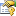﻿ LinearRegressionCoordinateDescent Class# LinearRegressionCoordinateDescent Class

Coordinate descent algorithm for the L1 or L2-loss linear Support Vector Regression (epsilon-SVR) learning problem in the dual form (-s 12 and -s 13).Inheritance Hierarchy
SystemObject
Accord.MachineLearning.VectorMachines.LearningBaseSupportVectorRegressionSupportVectorMachine, Linear, Double
Accord.MachineLearning.VectorMachines.LearningBaseLinearRegressionCoordinateDescentSupportVectorMachine, Linear, Double
Accord.MachineLearning.VectorMachines.LearningLinearRegressionCoordinateDescent

Namespace:  Accord.MachineLearning.VectorMachines.Learning
Assembly:  Accord.MachineLearning (in Accord.MachineLearning.dll) Version: 3.8.0Syntax
public class LinearRegressionCoordinateDescent : BaseLinearRegressionCoordinateDescent<SupportVectorMachine, Linear, double[]>

The LinearRegressionCoordinateDescent type exposes the following members.Constructors
NameDescriptionLinearRegressionCoordinateDescent
Initializes a new instance of the LinearRegressionCoordinateDescent class.LinearRegressionCoordinateDescent(SupportVectorMachine, Double, Double) Obsolete.
Obsolete.
TopProperties
NameDescriptionC
Gets or sets the cost values associated with each input vector.
(Inherited from BaseSupportVectorRegressionTModel, TKernel, TInput.)Complexity
Complexity (cost) parameter C. Increasing the value of C forces the creation of a more accurate model that may not generalize well. If this value is not set and UseComplexityHeuristic is set to true, the framework will automatically guess a value for C. If this value is manually set to something else, then UseComplexityHeuristic will be automatically disabled and the given value will be used instead.
(Inherited from BaseSupportVectorRegressionTModel, TKernel, TInput.)Epsilon
Insensitivity zone ε. Increasing the value of ε can result in fewer support vectors in the created model. Default value is 1e-3.
(Inherited from BaseSupportVectorRegressionTModel, TKernel, TInput.)Inputs
Gets or sets the input vectors for training.
(Inherited from BaseSupportVectorRegressionTModel, TKernel, TInput.)IsLinear
Gets whether the machine to be learned has a Linear kernel.
(Inherited from BaseSupportVectorRegressionTModel, TKernel, TInput.)Kernel
Gets or sets the kernel function use to create a kernel Support Vector Machine. If this property is set, UseKernelEstimation will be set to false.
(Inherited from BaseSupportVectorRegressionTModel, TKernel, TInput.)Lagrange
Gets the value for the Lagrange multipliers (alpha) for every observation vector.
(Inherited from BaseLinearRegressionCoordinateDescentTModel, TKernel, TInput.)Loss
Gets or sets the Loss cost function that should be optimized. Default is L2.
(Inherited from BaseLinearRegressionCoordinateDescentTModel, TKernel, TInput.)Model
Gets the machine to be taught.
(Inherited from BaseSupportVectorRegressionTModel, TKernel, TInput.)Outputs
Gets or sets the output values for each calibration vector.
(Inherited from BaseSupportVectorRegressionTModel, TKernel, TInput.)Token
Gets or sets a cancellation token that can be used to stop the learning algorithm while it is running.
(Inherited from BaseSupportVectorRegressionTModel, TKernel, TInput.)Tolerance
Convergence tolerance. Default value is 0.1.
(Inherited from BaseLinearRegressionCoordinateDescentTModel, TKernel, TInput.)UseComplexityHeuristic
Gets or sets a value indicating whether the Complexity parameter C should be computed automatically by employing an heuristic rule. Default is false.
(Inherited from BaseSupportVectorRegressionTModel, TKernel, TInput.)UseKernelEstimation
Gets or sets whether initial values for some kernel parameters should be estimated from the data, if possible. Default is true.
(Inherited from BaseSupportVectorRegressionTModel, TKernel, TInput.)Weights
Gets or sets the individual weight of each sample in the training set. If set to null, all samples will be assumed equal weight. Default is null.
(Inherited from BaseSupportVectorRegressionTModel, TKernel, TInput.)
TopMethods
NameDescriptionComputeError Obsolete.
Obsolete.
(Inherited from BaseSupportVectorRegressionTModel, TKernel, TInput.)Create
Creates an instance of the model to be learned. Inheritors of this abstract class must define this method so new models can be created from the training data.
(Overrides BaseSupportVectorRegressionTModel, TKernel, TInputCreate(Int32, TKernel).)Equals
Determines whether the specified object is equal to the current object.
(Inherited from Object.)Finalize
Allows an object to try to free resources and perform other cleanup operations before it is reclaimed by garbage collection.
(Inherited from Object.)GetHashCode
Serves as the default hash function.
(Inherited from Object.)GetType
Gets the Type of the current instance.
(Inherited from Object.)InnerRun
Runs the learning algorithm.
(Inherited from BaseLinearRegressionCoordinateDescentTModel, TKernel, TInput.)Learn
Learns a model that can map the given inputs to the given outputs.
(Inherited from BaseSupportVectorRegressionTModel, TKernel, TInput.)MemberwiseClone
Creates a shallow copy of the current Object.
(Inherited from Object.)Run Obsolete.
Obsolete.
(Inherited from BaseSupportVectorRegressionTModel, TKernel, TInput.)ToString
Returns a string that represents the current object.
(Inherited from Object.)
TopExtension Methods
NameDescriptionHasMethod
Checks whether an object implements a method with the given name.
(Defined by ExtensionMethods.)IsEqual
Compares two objects for equality, performing an elementwise comparison if the elements are vectors or matrices.
(Defined by Matrix.)To(Type)Overloaded.
Converts an object into another type, irrespective of whether the conversion can be done at compile time or not. This can be used to convert generic types to numeric types during runtime.
(Defined by ExtensionMethods.)ToTOverloaded.
Converts an object into another type, irrespective of whether the conversion can be done at compile time or not. This can be used to convert generic types to numeric types during runtime.
(Defined by ExtensionMethods.)
TopRemarks

This class implements a SupportVectorMachine learning algorithm specifically crafted for linear machines only. It provides a L2-regularized, L1 or L2-loss coordinate descent learning algorithm for optimizing the dual form of learning. The code has been based on liblinear's method solve_l2r_l1l2_svc method, whose original description is provided below.

Liblinear's solver -s 12: L2R_L2LOSS_SVR_DUAL and -s 13: L2R_L1LOSS_SVR_DUAL. A coordinate descent algorithm for L1-loss and L2-loss linear epsilon-vector regression (epsilon-SVR).

min_\beta  0.5\beta^T (Q + diag(lambda)) \beta - p \sum_{i=1}^l|\beta_i| + \sum_{i=1}^l yi\beta_i,
s.t.     -upper_bound_i <= \beta_i <= upper_bound_i,

where Qij = yi yj xi^T xj and D is a diagonal matrix

In L1-SVM case:

upper_bound_i = C
lambda_i = 0

In L2-SVM case:

upper_bound_i = INF
lambda_i = 1/(2*C)

Given: x, y, p, C and eps as the stopping tolerance

See Algorithm 4 of Ho and Lin, 2012.See Also